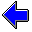Java Security Settings:
This web page employs Java, which requires specific security settings for correct operation.
If the applets on this page do not run correctly, consult the Virtual Chemistry Experiments FAQ
or the Physlet Physics web site for establishing the correct security settings.

# Gas Laws

## Calculations using Boyle's Law

### Concepts

Boyle's Law states that the product of the pressure and volume for a gas is a constant for a fixed amount of gas at a fixed temperature. Written in mathematical terms, this law is

P V = constant

A common use of this law is to predict how a change in pressure will alter the volume of the gas or vice versa. Such a problem can be regarded as a two state problem: the initial state (represented by subscript i) and the final state (represented by subscript f). If a sample of gas initially at pressure Pi and volume Vi is subjected to a change that does not change the amount of gas or the temperature, the final pressure Pf and volume Vf are related to the initial values by the equation

Pi Vi = Pf Vf

### Exercise

Objective

• Use Boyle's Law to predict the final pressure for a gas that has undergone an expansion.

In both parts of this exercise, you will work with two glass bulbs connected by a tube containing a stopcock. Initially, the bulb on the right is completely evacuated (that is, it contains no gas) while the bulb on the left contains a sample of gas at pressure Pi. A manometer is provided to measure the pressure in the left bulb.

When the stopcock is opened, the gas in the left bulb will expand to occupy both bulbs and the pressure in the left bulb will change accordingly. The temperature of the system is held constant throughout the experiment.

Information on the volumes of the two bulbs will be given to you, and you may read the initial pressure from the manometer. Use Boyle's Law to calculate the final pressure. Then open the stopcock and see if your prediction is correct.

Part 1

Each time you press the "New Conditions" button, the experiment will be reset with new volumes for the two bulbs and a new initial pressure. After calculating the final pressure, press the "Open Stopcock" button to allow the gas to expand and check your prediction.

Practice the calculations until you are able to solve the problem consistently.

 Volume of Left Bulb L Volume of Right Bulb L

Part 2

In performing the Boyle's Law calculations in Part 1, you will no doubt have observed that it is not necessary to know the initial and final volumes. It is only necessary to know the volume ratio: Vi/Vf.

This part of the exercise is the same as Part 1 except you are not given the volumes of the two bulbs. Instead you are given the ratio of the two volumes: Vright/Vleft.

Repeat the exercise using the volume ratio in the calculations. Bear in mind that Vright/Vleft is not the same as Vi/Vf .

 Vright/Vleft =Boyle's Law                     Charles's LawGas Laws Home PageVirtual Chemistry Home Page

BoylesLawCalc.html version 2.1